# Measurement error bias

## What is measurement error bias?

Errors-in-variables, or measurement error situation happens when your right hand side variable(s); your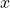in a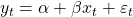model is measured with error. Ifrepresents the price of a liquid stock, then it is accurately measured because the trading is so frequent. But ifis a volatility, well, it is not accurately measured. We simply don’t yet have the power to tame this variable variable.

Unlike the price itself, volatility estimates change with our choice of measurement method. Since no model is a perfect depiction of reality, we have a measurement error problem on our hands.

Ignoring measurement errors leads to biased estimates and, good God, inconsistent estimates.

## What seems to be the problem officer?

Formally, if we think we are usingbut really we use are usingthen the usual regression model becomes:What we can see from the estimation ofin such a model, using the usual regression iswhich is the usual estimate for, but since we measure x with noiseUsing the usual covariance rules, and assuming the noise in x does has nothing to do with x itself we have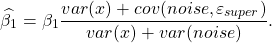Now if you assume the noise in x has nothing to do with the noise in y, meaningyou are left with a faulty estimate. Faulty in the sense that it is misleading; you are afterbut you get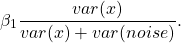How bad is your estimate depends on how accurately x is measured, the noisier, the stronger the bias. Actually, a more accurate statement is that the noisier it is, compared with the original variance of x, the larger the bias.

We don’t see the number of observations anywhere in this derivation so that’s bad news, since it means this bias is not going anywhere, not by adding more data at least.

The quantityis always smaller than 1. So our estimate is always weaker (smaller absolute value) than what it would otherwise be. This is called attenuation, or dilution. Why is this important? It matters for forecasting and for significance testing. Themay not be prominent enough to pass the test (because it is closer to zero than it should be), and we can continue working while wrongly dismissing that variable has having no real impact.The dashed line is the simulated parameter. The grey curve is the simulated distribution with no measurement errors. Notice how it is centered correctly. The green curve is simulated from an estimate with a ratio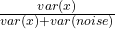ofand the blue curve is simulated from a ratio of.

You can see that then the ratio is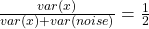the blue curve much more strongly attenuated. Something to keep in mind when making inference. Code is below.

### 2 comments on “Measurement error bias”

1.St says:

Isn’t the challenge figuring out estimates of “var(x)/(var(x)+var(noise))” in actual empirical data? (And not just assuming it)